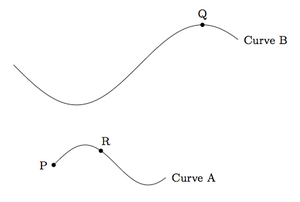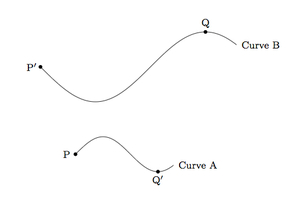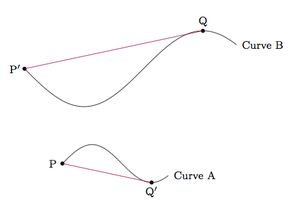# A scaled curve

Alignments to Content Standards: 8.G.A

Below are two curves with some labeled points.Curve B is a scaled version of curve A: this means that there is a scale factor $r \gt 0$ so that the distance between any pair of points on curve A is scaled by a factor of $r$ when looking at the corresponding points on curve B.

1. Identify and label the point on curve B corresponding to the point P on curve A. How do you know you have chosen the correct point?
2. Identify and label the point on curve A corresponding to the point Q on curve B. How do you know you have chosen the correct point?
3. Take measurements to find the scale factor going from curve A to curve B? Explain.
4. Can you find the point on curve B corresponding to the point R on curve A? Explain.

## IM Commentary

The goal of this task is to motivate and prepare students for the formal definition of dilations and similarity transformations. While these notions are typically applied to triangles and quadrilaterals, having students engage with the concepts in a context where they don't have as much training (these more "random" curves) lead students to focus more on the properties of the transformations than the properties of the figure.

The first two parts of the task are aligned with 7.G.1 and then the second two parts help to motivate the need for a more formal definition of similarity which is a key ingredient to 8.G.A. Students will already be comfortable with what it means to scale line segments and polygons (same shape but possibly different size). In order to motivate the technical and abstract notion of similarity transformations, the objects in this task are chosen to be complex curves. Geometric intuition is still valuable here as is seen in parts (a) and (b). Parts (c) and (d) are far more challenging. Since the shape is curved, finding the scale factor is more challenging than for the case of polygons because it is not easy to measure the length of the curves and some other method is needed. This part of the task brings out one very important aspect of similarity transformations, namely that they scale distances of all points in the plane in a uniform way: this allows us to find the scale factor by looking at two pairs of corresponding points and measuring the distance between them.

The final part of the task is very challenging in the absence of a precise working definition of similarity. Students should be able to find the ''approximate'' point on B that corresponds to R via qualitative reasoning: "if I trace curve B starting at P$^\prime$ I need to go past the first hump but not too far past.'' They can gain better accuracy by taking measurements and using their findings from (c). In fact, if they are sufficiently creative, they can find a plausible method for identifying the point with some creativity. It is also important to note that if we were not given curve B, then the method at the end of the solution would not be adequate to identify the point corresponding to R on curve A: the circles in the construction meet at two different points and so more work would be needed to make the correct choice.

With the formal definition of similarity transformation, however, students can answer part (d) in steps as follows:

• Apply the scale factor found in part (c) to curve A.
• Apply translations, rotations, and/or reflections (either with patty paper or with more formal constructions) in order to move the scaled version of curve A so that it corresponds point by point with curve B. This will tell us which point on curve B corresponds to R.

Without the formal definition of similarity transformations or the construction with circles, there is another important method students can employ to estimate the point on curve B corresponding to R. The student can estimate the length of curve A from P to R by choosing several points between P and R on curve A and summing the lengths of the segments joining consecutive points. The same technique can be applied on curve B, namely adding many points and measuring the length of line segments gives us a way of finding a point on curve B whose distance from P$^\prime$ along the curve is close to a chosen value. Choosing as our value the approximate distance along curve A from P to R, multiplied by the scale factor going from curve A to curve B, will give a point on curve B which approximately corresponds to R. The more line segments we add, the better the correspondence will become.

## Solution

1. The point P is one of the two endpoints of curve $A$ and so the corresponding point P$^\prime$ of curve B will be one of its two endpoints. In order to determine which one, notice that P is the endpoint further from the nearest peak or valley of curve A. The distances of the endpoints from the nearest peak and valley are both scaled by the same positive factor $r$. This means that P$^\prime$ must be the endpoint of curve B furthest from a peak or valley. It is marked below:2. Following the reasoning in part (a), the point Q on curve B is the peak or valley furthest from the point P$^\prime$. So the corresponding point on curve A is the point marked Q$^\prime$ below:3. We usually find the scale factor by measuring corresponding segments of our one dimensional shapes. For these two curves, this is not possible because they do not have any straight parts to measure. On the other hand, we have a pair of corresponding points on the two curves and so when the curves are scaled by a factor of $r$, the segments joining those corresponding pairs of points will also be scaled by a factor of $r$. These segments are shown below:We can find the scale factor by measuring these corresponding segments and taking the quotient. This means that the scale factor going from curve A to curve B is $$\frac{\left|P^\prime Q\right|}{\left|PQ^\prime \right|}.$$ Measuring these lengths, the scale factor going from curve A to curve B appears to be about 2.

4. Below we plot point R on curve A and make a triangle with our three points P, Q$^\prime$, and R:The point R$^\prime$ on Curve B corresponding to R on curve A will have the property that $$\frac{\left|P^\prime R^\prime \right|}{\left|R^\prime Q\right|}=\frac{\left|P R \right|}{\left|R Q^\prime\right|}$$ because scaling a picture maintains internal proportions. Using this information we can experiment and find a good approximation for the point R$^\prime$ on curve B that corresponds to point R.

Alternatively, since we know the scale factor going from curve A to curve B we know how far R$^\prime$ is from $\text{P}^\prime$ and how far it is from Q. This information is enough to determine where R$^\prime$ is on curve B. Below is a picture showing a way to do this:The radius of the circle with center P$^\prime$ is twice $|PR|$ while the circle with center $Q$ has radius twice $\left|RQ^\prime\right|$. The point where they meet on curve B corresponds to point $R$ on Curve A. Since each of these circles meets curve B in only one point, we do not need both in order to identify R$^\prime$: it is good practice, however, to check that $|P^\prime R^\prime| = 2|PR|$ and $|R^\prime Q| = 2| RQ^\prime|$.